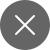# Cross-Validation in Machine learning

7+

You always have to validate your machine learning model. In machine learning, you cannot just fit the model on the training data and say it will work for real-world data too. You have to be confident that your model got well-versed with the patterns in the data correctly and not getting too much noise. To build this confidence you have to use something called Cross-validation. In this article, we will get a proper idea of cross-validation and it’s working.

Also, check about – confusion matrix and cross-entropy loss

# What is cross-validation?

Cross-validation is a method to evaluate the performance of a machine learning model. It is done by training the model on a subset of input data and testing on the unseen subset of data.

The main aim of cross-validation is to estimate how the model will perform on unseen data. It is easy to understand, implement, and possess lower bias when compared to other methods used for measuring the model’s performance. Let’s take an example, to elaborate on this. Suppose a child is learning to ride a bicycle. Now he can easily ride the bicycle on an empty road. However, the actual challenge is when he is riding in traffic. That is why you have to train him on roads having roads to make him used to it. Now, the child will be able to ride a bicycle without any assistance. This is how our cross-validation technique also works.

Let’s see the basic steps of cross-validation –

1. Reservation of a subset of given data for validation
2. Train the model using the training dataset
3. Evaluate the model’s performance using the validation dataset

# Different techniques for Cross-validation

Now, we are going to discuss commonly used methods for cross-validation  They are as follows:

• Validation
• Leave one out cross-validation
• Leave-P-out cross-validation
•  K-fold cross-validation
• Stratified K-fold cross-validation

## Validation

In this approach, we will split our dataset into a training set and testing set. Training is performed on 50% of the given dataset and the remaining 50% is used to test our model. The main limitation of this approach is that we are using only 50% of the data to train our model. It may be a case that the remaining 50% dataset has some useful information that our model is missing. Sometimes it may lead to underfitting too.

Implement :

LOOCV (Leave one out cross-validation)

In this approach of cross-validation, the complete dataset is used for training except for one data point of the given dataset. They will keep iterating for all samples in the dataset. You can choose this approach for a small dataset. This approach gives low bias as we consider all the data points here. Although, as the validation process is being repeated ‘n’ several times (where n is the number of data points), it results in greater execution time.

Leave-P-out cross-validation

This approach is similar to LOOCV In this approach if you’re having k data points then k-p data points will be used for training, and p data points are used for testing. This complete procedure will be repeated for all the samples. Then, we will calculate the average error to estimate our model’s performance.

When you will impute p = 1, it will result in LOOCV. It is less computational when compared to LOOCV.

## K-fold cross-validation

In this approach, the given dataset will be divided into K parts (folds) of equal sizes. In this approach, k-1 data points are used for training our model and the remaining data point is used for validation purposes.  In this technique, we will iterate k times with a particular subset reserved for validation purposes each time. It is a very popular cross-validation technique because it is very easy to grasp and implement. Also, the bias is very less when compared to other approaches. To calculate the effectiveness of the model, we have to average the error estimation of all K-folds. Generally, we prefer the value of K as 5 or 10 but it’s not fixed. You can choose any value.

In the below, we have taken an example of 5-folds cross-validation. We can see that in the first iteration, the first block is reserved for testing. On the second iteration, the second block got reserved for testing, and this how it continues up to the 5th iteration.

Implement:

## Stratified K-fold cross-validation

This technique is comparable to k-fold cross-validation with some little changes. The method of rearranging the dataset to make sure that every fold is a  good representative of the complete dataset is termed stratification. To handle the bias and variance, it’s one in all the simplest approaches.

For instance, within the case of a binary classification problem, each class comprises 50% of the data. To Illustrate the ratio is 30% and 70% distribution. The best practice is to rearrange the information so each class consists of the identical 30% and 70% distribution in every fold. The stratification process is best suited to small and unbalanced datasets with multiclass classification.

This was pretty much about cross-validation and its types. let’ see the advantages of cross-validation.

1. It helps in reducing overfitting.
2. It helps in calculating the optimal values of hyperparameters which results in increasing the efficiency of the algorithm.
3. Data is used efficiently as all samples are used for training and testing.

1. Training time increases as a model goes through multiple iterations for the given dataset.
2. Computation power increases.7+

### 2 Responses

1. December 26, 2020

[…] check about cross-validation and cross-entropy […]

0
2. December 30, 2020

[…] check about cross-validation and cross-entropy […]

0